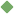regression 1. [ noun ] (mathematics,statistics) the relation between selected values of x and observed values of y (from which the most probable value of y can be predicted for any value of x) Synonyms: regression_toward_the_mean statistical_regression simple_regression Related terms: statistics statistical_method curvilinear_regression linear_regression regression_analysis To share this definition press "text" (Facebook, Twitter) or "link" (blog, mail) then paste text link 2. [ noun ] (psychiatry) a defense mechanism in which you flee from reality by assuming a more infantile state Related terms: defense psychiatry 3. [ noun ] (medicine) an abnormal state in which development has stopped prematurely Synonyms: fixation infantile_fixation arrested_development Related terms: abnormality 4. [ noun ] returning to a former state Synonyms: reversion retrogression retroversion regress Related terms: reversal revert Similar spelling: regress   regressing   regressive   recursion